# Competition Level Test: Relations And Functions- 2

## 30 Questions MCQ Test Mathematics (Maths) Class 11 | Competition Level Test: Relations And Functions- 2

Description
This mock test of Competition Level Test: Relations And Functions- 2 for JEE helps you for every JEE entrance exam. This contains 30 Multiple Choice Questions for JEE Competition Level Test: Relations And Functions- 2 (mcq) to study with solutions a complete question bank. The solved questions answers in this Competition Level Test: Relations And Functions- 2 quiz give you a good mix of easy questions and tough questions. JEE students definitely take this Competition Level Test: Relations And Functions- 2 exercise for a better result in the exam. You can find other Competition Level Test: Relations And Functions- 2 extra questions, long questions & short questions for JEE on EduRev as well by searching above.
QUESTION: 1

### Let  A = {1, 2, 3} and let R = {(1, 1), (2, 2), (3, 3), (1, 3), (3, 2), (1, 2)}. Then R is

Solution:

R is reflexive and transitive but not symmetric

QUESTION: 2

### Let A {a, b, c} and let R = {(a, a)(a, b), (b, a)}. Then, R is

Solution:

R is symmetric and transitive but not reflexive

QUESTION: 3

### Let A = {1, 2, 3} then total number of element in A x A is

Solution:
QUESTION: 4

Let S be the set of all straight lines in a plane. Let R be a relation on S defined by a R b ⇔ a ⊥ b. then, R is

Solution:

a ⊥ a is not true. So, R is not reflexive
a ⊥ b and b ⊥ c does not imply a ⊥ c. So, R is not transitive
But, a ⊥ b ⇒ b ⊥ a is always true.

QUESTION: 5

Let S be the set of all real numbers and let R be a relation on S, defined by a R b ⇔ |a – b| < 1. Then, R is

Solution:

(i) |a – a| = 0 < 1 is always true
(ii) a R b ⇒ |a – b| < 1 ⇒ |-(a – b)| < ⇒ |b – a| < 1 ⇒ b R a.
(iii) 2R 1 and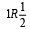But, 2 is not related to 1/2. So, R is not transitive.

QUESTION: 6

Domain of f (x)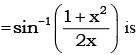Solution:

Domain of sin -1x is [-1,1]

QUESTION: 7

Let R be the relation in the set N given by R = {(a, b): a = b – 2, b > 6}. Choose the correct answer.

Solution:

R = {(a, b): a = b − 2, b > 6}
Now, since b > 6, (2, 4) ∉ R
Also, as 3 ≠ 8 − 2, (3, 8) ∉ R
And, as 8 ≠ 7 − 2
∴ (8, 7) ∉ R
Now, consider (6, 8).
We have 8 > 6 and also, 6 = 8 − 2.
∴ (6, 8) ∈ R

QUESTION: 8

Let R = {(3, 3), (6, 6), (9, 9), (3,6), (3, 9), (9, 12), (3,12), (6, 12), (12, 12)}, be a relation on the set A = {3, 6, 9, 12} Then the relation is

Solution:

R is reflexive
∴ (3, 3), (6,6), (9, 9), (12, 12) ∈ R
again ∴ (6, 12) ∈ R but (12, 6) ∉ R ⇒ R is not symmetric
R is transitive
[∴ (3, 6) ∈ R, (6, 12) ∈ R, (3, 12) ∈ R others are clear

QUESTION: 9

If f(x) = (a – xn)1/n.  then f(f(x)) =

Solution:

f(x) = (a – xn)1/n = y

QUESTION: 10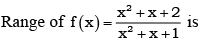Solution:
QUESTION: 11

f: N → N : f(x) = 2x is

Solution:

The function is injective (one-to-one) if every element of the codomain is mapped to by at most one element of the domain

The function is surjective (onto) if every element of the codomain is mapped to by at least one element of the domain

f(x)=2x

Domain of f is N

Codomain of f is N

Every element of N(codomain) is mapped to only one element in N(domain)

i.e. f(x)=f(y)

⟹2x=2y⟹x=y

Hence f is one one function

Every even element of N(codomain) has corresponding value in N(domain).

But, for any odd number in N(codomain) has no corresponding value in Ndomain.

Hence, f is not onto function.

QUESTION: 12

f: R → R : f(x) = x2 is

Solution:

Given, function f : R→R such that f(x) = x2
Let A and B be two sets of real numbers.
Let x1, x2 ∈ A such that f(x1) = f(x2).
⇒1 + (x1)2 = 1 + (x2)2
​⇒ (x1)2 − (x2)2 = 0
⇒(x1 − x2)(x1 + x2)=0
⇒ x1 = ± x2
Thus f(x1) = f(x2) does not imply that x1 = x2.
For instance, f(1) = f(−1) = 1, i.e. , two elements (1, -1) of A have the same image in B. So, f is many-one function.

QUESTION: 13

f : R → R : f(x) = x3 is

Solution: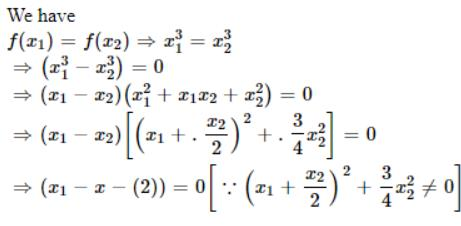⇒ x2 = x2

∴ f is one-one

Let y∈R and let y=x3. then x=y ∈ R

Thus for each y in the codomain R there exists y1/3 in R such that

f(y13)=(y13)3=y

∴ f is onto.

Hence f is one -one onto.

QUESTION: 14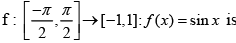Solution:

f(x) = sinx
f(x) = sinx is a one-one function.
codomain = range
therefore, f(x) = sinx is an onto function.

QUESTION: 15

f : R → R : f(x) = cos x is

Solution:

cos(2π - θ) = cosq ⇒ f is many-one.
Range (f) = [-1,1] ⊂ R ⇒ f is in to.

QUESTION: 16

The domain of the function f = {(1, 3), (3, 5), (2, 6)} is

Solution:

Domain = {1, 3, 2}

QUESTION: 17

Let f(x) = x - 1/x +1 , x ≠ -1, then f-1 (x) is

Solution:
QUESTION: 18

If f(x) = x/x -1 , x ≠ 1, then f-1 (x) is

Solution:
QUESTION: 19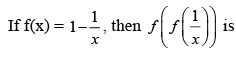Solution:
QUESTION: 20

If f(x) = cos (log x), then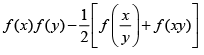has the value

Solution: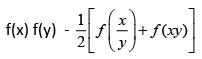= cos (log x) cos (cos y)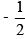[cos(log(x/y))+cos(log(xy))]
= cos (log x) cos (log y)[cos(log x - log y)+cos(log x +log y)]
= cos (log x) cos (log y)[2 cos(log  x)cos(log y)] = 0

QUESTION: 21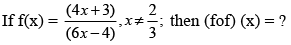Solution: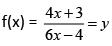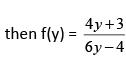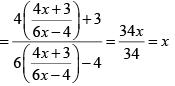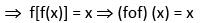QUESTION: 22

If f(x) =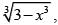then (fof) (x) = ?

Solution:

(fof) (x) = f[f(x)] – {(3 – x3)1/3} = f(y)  where y = (3 – x3)1/3
= (3 – y3)1/3 = [3- (3 – x3)]1/3 = (x3)1/3 = x

QUESTION: 23

If f(x) = x2 – 3x + 2, then (fof) (x) = ?

Solution:

(fof) (x) = f[f(x)] = f(x2 - 3x + 2)2 - 3(x2 – 3x + 2)
= y2 – 3y + 2 = (x2 – 3x + 2)2 – 3(x2 – 3x + 2) + 2 =  (x4 – 6x3 + 10x2 – 3x)

QUESTION: 24

Let S = {1, 2, 3}. The function f : S → S defined as below have inverse for

Solution:

Since f(2) = f(3) = 1, then inverse does not exists
Inverse exist for (C) f-1 = {(3, 1), (2, 3), (1, 2)}.

QUESTION: 25

The relation R defined on the set N of natural numbers by xRy ⇔2x2 - 3xy + y2 = 0 is

Solution:

(i) xRx ⇔ 2x2 - 3x.x + x2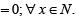∴ R is reflexive
(ii) For x = 1, y= 2; 2x2 - 3xy + y2 = 0
∴ 1 R 2 but 2.22 - 3.2.1 + 12 = 3 ≠ 0.
So, 2 is not R-related to 1.
∴ R is not symmetric.

QUESTION: 26

Let R = {(3, 3), (6, 6), (9, 9), (12, 12), (6, 12), (3, 9), (3, 12), (3, 6) be a relation on the set A = {3, 6, 9, 12}. The relation is

Solution:

Since, (3, 3), (6, 6), (9, 9), (12, 12) ∈ R ⇒ R is reflexive relation.
Now, (6, 12)∉R but (12, 6) ∉ R  ⇒ R is not a symmetric relation.
Also, (3, 6),(6, 12) ∈ R ⇒ (3, 12) ∈ R
⇒ R is transitive relation.

QUESTION: 27

Let R be a relation on the set A of ordered pairs of positive integers defined by (x, y)R(u, v) if and only if xv = yu, then

Solution:

Clearly, (x, y)R(x, y),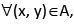since xy = yx. This shows that R is reflexive.
Further, (x, y)R(u, v) ⇒ xv = yu ⇒ uy vx and hence (u, v)R(x, y). This shows that R is symmetric.
Similarly, (x, y)R(u, v) and (u, v)R(a, b) ⇒ xv = yu and ub = va ⇒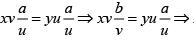xb = ya and hence (x, y)R(a, b). Thus, R is transitive. Thus, R is an equivalent relation.

QUESTION: 28

If R be a relation defined as aRb iff |a -b|> 0, then the relation is

Solution:

Since, R is a defined as aRb iff |a – b| > 0
For reflexive aRa iff |a – a| > 0
Which is not true, So, R is not reflexive
For symmetric aRb iff |a – b| > 0
Now bRa iff |b – a| > 0
⇒ |a – b| > 0  ⇒ ArB
Thus, R is symmetric.
For transitive aRb iff |a – b| > 0
bRc iff |b – c| > 0
⇒ |a – b + b – c| > 0
⇒ |a – c| > 0 ⇒ | c – a| > 0 ⇒ aRc
∴ R is transitive

QUESTION: 29

If R is an equivalence relation of a set A, then R-1 is

Solution:

If R is an equivalence relation, then R-1 is also an equivalence relation.

QUESTION: 30

Let r be a relation from R (set of real numbers) to R defined by r = {(a, b)|a, b∈R and a - b + √3 is an irrational number}. The relation r is

Solution:

Given, r = {(a, b)| a, b∈R and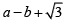as an irrational number
(i) Reflexive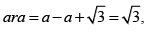which is irrational number.
(ii) Symmetric
Now,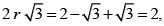which is not an irrational.
Also,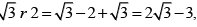which is an irrational.
Which is not symmetric.
(iii) Transitive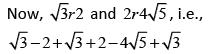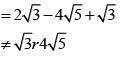∴ It is not transitive.